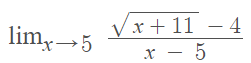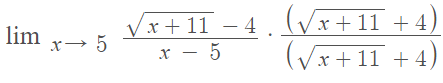# Conjugate Method for Limits: Definition, Examples

## What is the Conjugate Method for Limits?

The conjugate method is used for evaluating limits that contain radicals (√). Conjugates are particularly useful in evaluating some limits because they allow us to eliminate radicals. Due to its limited scope, you should use this method after you have tried the direct substitution method. If the substitution method results in an indeterminate limit like 0/0, try the conjugate method to see if it works.

## What is a “Conjugate”?

A conjugate is a binomial expression with opposite middle signs (a binomial is the sum or the difference of two terms). The “middle sign” separates the radical part of the expression from a constant. In other words, each pair has identical terms, differing only by the sign separating them.

Don’t change all of the signs in the expression—only the change the sign separating the radical from the constant. This can get a bit confusing if you have multiple signs in an expression. A few examples to help clear this up:

 If you have this in your formula …use this Conjugate √(x – 5) √(x + 5) √(x + 5) √(x – 5) √(x + 11) – 4 √(x + 11) + 4 2√(2) – 1 2√(2) + 1

Using conjugates in an expression is really useful because they work to eliminate radicals. Let’s say you had a non-radical expression like 4x = 8. In order to eliminate the “4”, you would divide by 4:

• 4x/4 = 8/4 → x = 2

In the same way, you use a conjugate to eliminate a radical. That’s because the product of two conjugates containing radicals will equal a result with no radicals. For example:

• √(x – 5) * √(x + 5)
• = √x2 + 5√(x) – 5√(x) – 25
• = x – 25

## Conjugate Method for Limits: Examples

Example problem #1: Solve the following limit using the conjugate method:This first example doesn’t work with substitution. Try it! Substitute in x = 5 into the expression and you’ll get an indeterminate limit (0/0).

Step 1: Multiply the numerator and the denominator by the conjugate:Step 2: Simplify, using algebra:

1.2.3.Step 3: Evaluate the limit at the given point (for this example, that’s x = 5).Example problem #2: Evaluate the following limit:Step 1: Multiply the numerator and the denominator by the conjugate:Step 2: Simplify, using algebra:

1.2.3.Step 3: Evaluate the limit at the given point (for this example, that’s x = 4).CITE THIS AS:
Stephanie Glen. "Conjugate Method for Limits: Definition, Examples" From StatisticsHowTo.com: Elementary Statistics for the rest of us! https://www.statisticshowto.com/conjugate-method-for-limits-definition-examples/